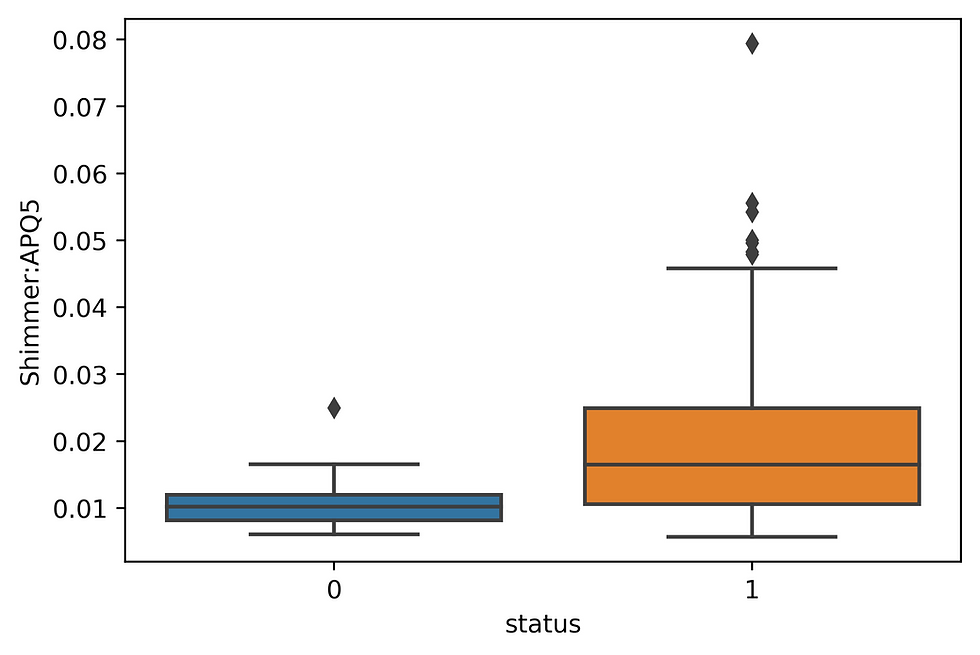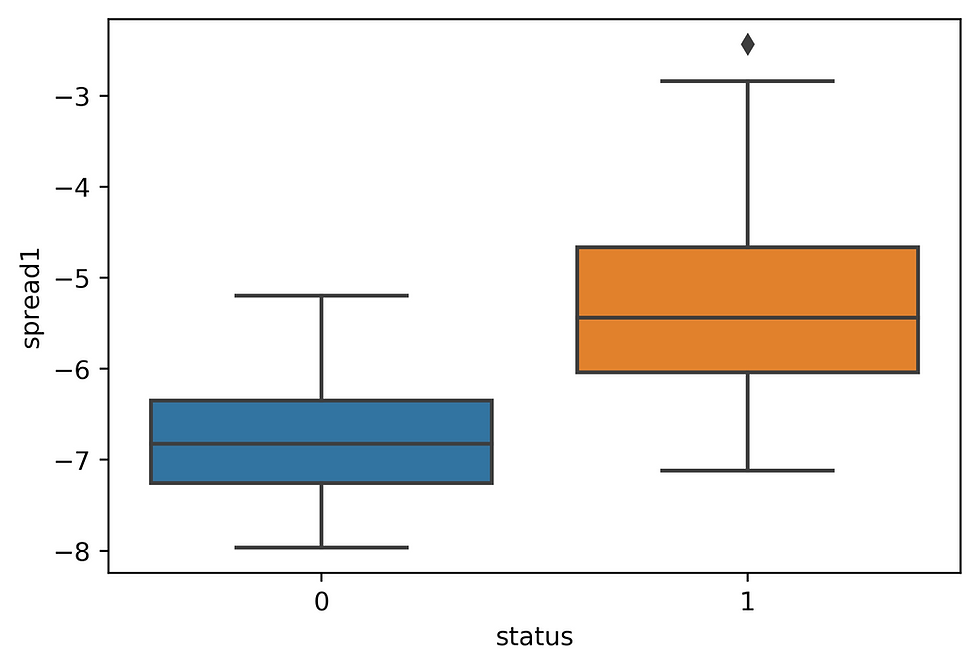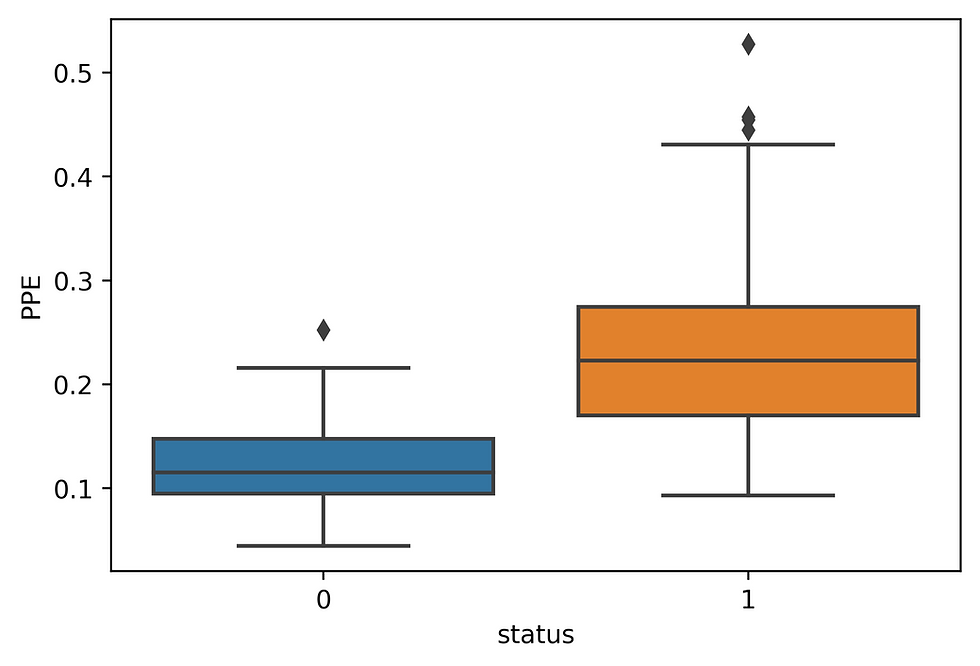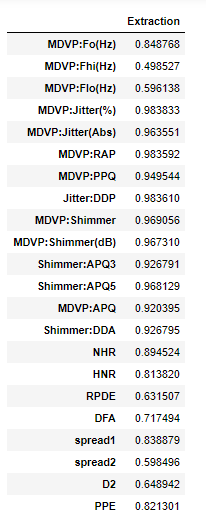top of page

# FACTOR ANALYSIS AND PREDICTION OF PARKINSON'S DISEASES

Neurodegenerative diseases are a heterogeneous group of disorders that are characterized by the progressive degeneration of the structure and function of the nervous system. They are incurable and debilitating conditions that cause problems with mental functioning also called dementia.

Neurodegenerative diseases affect millions of people world wide. Alzheimer’s disease and Parkinson’s disease are the most common neurodegenerative diseases. In 2016, an estimated 5.4 million Americans were living with Alzheimer’s disease. An estimated 930,000 people in the United States could be living with Parkinson’s disease by 2020.

Data Set Information:

This data set was created by Max Little of the University of Oxford, in collaboration with the National Center for Voice and Speech, Denver,

Colorado, who recorded the speech signals. Data Set Used for analysis and prediction is available at UCI ML Parkinson’s

Attribute Information:

name - ASCII subject name and recording number

MDVP:Fo(Hz) - Average vocal fundamental frequency

MDVP:Fhi(Hz) - Maximum vocal fundamental frequency

MDVP:Flo(Hz) - Minimum vocal fundamental frequency

MDVP:Jitter(%), MDVP:Jitter(Abs), MDVP:RAP, MDVP:PPQ, Jitter:DDP - Several measures of variation in fundamental frequency

MDVP:Shimmer, MDVP:Shimmer(dB), Shimmer:APQ3, Shimmer:APQ5, MDVP:APQ, Shimmer:DDA - Several measures of variation in amplitude

NHR, HNR - Two measures of ratio of noise to tonal components in the voice

status - Health status of the subject (one) - Parkinson's, (zero) - healthy

RPDE, D2 - Two nonlinear dynamical complexity measures

DFA - Signal fractal scaling exponent

```#Importing data exploration and visualization packages
import numpy as np
import pandas as pd
import seaborn as sns
import matplotlib.pyplot as plt```
```# Reading data from csv file into dataframe

Exploring Data Set

`df.head()``df.shape`

(195, 24)

`df.info()`

<class 'pandas.core.frame.DataFrame'> RangeIndex: 195 entries, 0 to 194 Data columns (total 24 columns): name 195 non-null object MDVP:Fo(Hz) 195 non-null float64 MDVP:Fhi(Hz) 195 non-null float64 MDVP:Flo(Hz) 195 non-null float64 MDVP:Jitter(%) 195 non-null float64 MDVP:Jitter(Abs) 195 non-null float64 MDVP:RAP 195 non-null float64 MDVP:PPQ 195 non-null float64 Jitter:DDP 195 non-null float64 MDVP:Shimmer 195 non-null float64 MDVP:Shimmer(dB) 195 non-null float64 Shimmer:APQ3 195 non-null float64 Shimmer:APQ5 195 non-null float64 MDVP:APQ 195 non-null float64 Shimmer:DDA 195 non-null float64 NHR 195 non-null float64 HNR 195 non-null float64 status 195 non-null int64 RPDE 195 non-null float64 DFA 195 non-null float64 spread1 195 non-null float64 spread2 195 non-null float64 D2 195 non-null float64 PPE 195 non-null float64 dtypes: float64(22), int64(1), object(1) memory usage: 36.6+ KB

```# Showing Summary Statistics
df.describe()```Bi-variate Analysis:

```# Extracting Column names from the data set
columns = list(X.columns.values)

# Plot Boxplots for the bivariate analysis on all columns
for column in columns:
sns.boxplot(y, column, data=df)
plt.show() ```Diagonal Correlation Matrix:

```# Creating Diagonal Correlation Matrix Using seaborn
corr = df.corr()

# Set up the matplotlib figure
f, ax = plt.subplots(figsize=(15, 15))

# Generate a custom diverging colormap
cmap = sns.diverging_palette(220, 10, as_cmap=True)

# Draw the heatmap with the mask and correct aspect ratio
square=True, linewidths=.5, cbar_kws={"shrink": .5})
plt.show()

```Factor Analysis:

```# Importing Factor Analyzer
from factor_analyzer import FactorAnalyzer```
1. Perform Bartlett's Test

```# Checking if the data is reliable for factor analysis
from factor_analyzer.factor_analyzer import calculate_bartlett_sphericity, calculate_kmo

chi_square_value, p_value = calculate_bartlett_sphericity(X)
print("Bartlett's Test of Sphericity Result is: {} with p_value: {}".format(chi_square_value, p_value))```

Bartlett's Test of Sphericity Result is: 13446.360031984032 with p_value: 0.0

Bartlett’s test of sphericity checks whether or not the observed variables intercorrelate at all using the observed correlation matrix against the identity matrix. If the test found statistically insignificant, you should not employ a factor analysis. Statistically insignificant means p_value greater than 0.05. In this case p_value is smaller than 0.05 so we can perform factor analysis.

2. Perform KMO Test

```# Checking if we sufficient data for factor analysis
kmo_all, kmo_model = calculate_kmo(X)
print("KMO Test Result is: {}".format(kmo_model))```

KMO Test Result is: 0.781509980596122

Kaiser-Meyer-Olkin (KMO) Test measures the suitability of data for factor analysis. It determines the adequacy for each observed variable and for the complete model. KMO estimates the proportion of variance among all the observed variable. Lower proportion id more suitable for factor analysis. KMO values range between 0 and 1. Value of KMO less than 0.7 is considered inadequate.

3. Getting eigen-values to Determine Number of Factors

```# Fitting the Factor Analyzer and Printing Resulting eigen-values numpy array
fa = FactorAnalyzer(rotation=None)
fa.fit(X)

# Check Eigenvalues
ev, v = fa.get_eigenvalues()
ev```

array([12.958111, 2.485875, 1.542030, 1.464986, 0.973916, 0.729108, 0.552245, 0.362403, 0.289838, 0.224126, 0.140565, 0.104841, 0.069737, 0.038166, 0.022012, 0.017788, 0.012456, 0.007214, 0.003497, 0.001085, 0.000000, 0.000000])

4. Scree Plot to Determine Number of Factors

```plt.scatter(range(1, X.shape+1),ev)
plt.plot(range(1, X.shape+1),ev)
plt.title('Scree Plot')
plt.xlabel('Factors')
plt.ylabel('Eigenvalue')
plt.grid()
plt.savefig('Scree Plot.jpg', dpi=500, bbc_inches='tight')
plt.show()```Scree Plot and Kaiser's eigen-values method both are used to determine the number of factors. In both the cases we look for a eigen-value greater than 1. The number of eigen-values greater than 1 are the number of factors that should be considered. In our case number of factors are 4 which is clearly visible from the data.

5. Fitting Factor Analyzer Using Determined No. of Factors

```# Selecting 4 factors as there are 4 eigen values that are above 1
fa = FactorAnalyzer(n_factors=4, method='principal', rotation='varimax')
fa.fit(X)

```

Select the method to use for factor analysis and rotation technique. There are 3 methods for factor analysis and several techniques of rotation available in factor analyzer package according to documentation.

In this case used principal component method and varimax rotation technique with n_factors equal to the values determined by using scree plot and Kaiser's eigen-value method.

6. Check Extraction Value in Communalities

```# Getting communalities of the factors
communalities = fa.get_communalities()
pd.DataFrame(data=communalities, index=columns, columns=['Extraction'])```Remove those variables from analysis that have communalities Extraction value less than 0.5. And start performing analysis from step 4. Factor Analysis is an iterative process you keep repeating it until no Extraction smaller than 0.5 is in communalities.

```# Showing which variable loads on which factor8. Check the variance explained by the factors

```# Getting the variance explained by the factors
variance = np.asarray(fa.get_factor_variance())

pd.DataFrame(data=variance, index=["Variance", "Proportional Variance", "Cumulative Variances"], columns=['Factor1','Factor2','Factor3','Factor4'])

```In our case 4 factors have cumulative variance of 0.838682 which is around 84% of variance.

Performing PCA Analysis to Show Total Variance Explained By 4 Factors:

```from sklearn.decomposition import PCA
from sklearn.preprocessing import StandardScaler```
```# Scaling Data before doing pca
scaler = StandardScaler()
X1 = scaler.fit_transform(X)

# Performing
pca = PCA(n_components = 4)
X_decomposed = pca.fit_transform(X1)

explained_variance = pca.explained_variance_ratio_

print('Total variance explained by 4 factors of PCA: {}'.format(explained_variance.sum()))```

Total variance explained by 4 factors of PCA: 0.8386818850900144

Which is same as obtained by the Factor Analysis that is because we used 'principal' method of factor analysis.

Importing Libraries for Predictive Modeling:

```from sklearn.model_selection import train_test_split
from sklearn.metrics import accuracy_score, f1_score
from sklearn.linear_model import LogisticRegression
from sklearn.tree import DecisionTreeClassifier
from sklearn.svm import SVC
```# Splitting data into Training and Testing Set
X_train, X_test, y_train, y_test = train_test_split(X_decomposed, y, test_size=0.3, random_state=0)```

Splitting data in training and test sets in 70-30 ratio.

Fitting Different Models And Making Predictions:

```# Fitting Logistic Regression Model for training
lr = LogisticRegression(solver='liblinear')
lr.fit(X_train, y_train)

y_pred = lr.predict(X_test)

print("The accuracy of Logistic Regression Model is {}".format(accuracy_score(y_test, y_pred)))
print("The f1-score of Logistic Regression Model is {}".format(f1_score(y_test, y_pred)))```

The accuracy of Logistic Regression Model is 0.8813559322033898 The f1-score of Logistic Regression Model is 0.924731182795699

```# Fitting Decision Tree Classifier Model for training
dt = DecisionTreeClassifier()
dt.fit(X_train, y_train)

y_pred = dt.predict(X_test)

print("The accuracy of Decision Tree Model is {}".format(accuracy_score(y_test, y_pred)))
print("The f1-score of Decision Tree Model is {}".format(f1_score(y_test, y_pred)))

```

The accuracy of Decision Tree Model is 0.9322033898305084 The f1-score of Decision Tree Model is 0.9583333333333334

```# Fitting Support Vector Classifier Model for training
sv = SVC(gamma='scale')
sv.fit(X_train, y_train)

y_pred = sv.predict(X_test)

print("The accuracy of Support Vector Model is {}".format(accuracy_score(y_test, y_pred)))
print("The f1-score of Support Vector Model is {}".format(f1_score(y_test, y_pred)))```

The accuracy of Support Vector Model is 0.9322033898305084 The f1-score of Support Vector Model is 0.9583333333333334

```# Fitting Gradient Boosting Classifier Model for training
gb.fit(X_train, y_train)

y_pred = gb.predict(X_test)

print("The accuracy of Gradient Boosting Model is {}".format(accuracy_score(y_test, y_pred)))
print("The f1-score of Gradient Boosting Model is {}".format(f1_score(y_test, y_pred)))```

The accuracy of Gradient Boosting Model is 0.9661016949152542 The f1-score of Gradient Boosting Model is 0.9777777777777777

```# Fitting Random Forest Classifier Model for training
rf = RandomForestClassifier(n_estimators=10)
rf.fit(X_train, y_train)

y_pred = rf.predict(X_test)

print("The accuracy of Random Forest Model is {}".format(accuracy_score(y_test, y_pred)))
print("The f1-score of Random Forest Model is {}".format(f1_score(y_test, y_pred)))```

The accuracy of Random Forest Model is 0.9152542372881356 The f1-score of Random Forest Model is 0.9438202247191011

Gradient Booting Classifier is the best model to use for predicting Parkinson's diseases.